JMSLTM Numerical Library 7.2.0
com.imsl.stat

## Class ANCOVA

• All Implemented Interfaces:
Serializable, Cloneable

```public class ANCOVA
extends Object
implements Serializable, Cloneable```
Analyzes a one-way classification model with covariates. Class ``` ANCOVA``` performs analysis for models that combine the features of a oneway analysis of variance model with that of a multiple linear regression model. The basic one-way analysis of covariance model is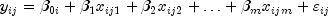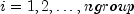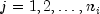where, ngroup is the number of treatment groups, the observed value of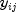constitutes the j-th response in the i -th group,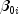denotes the y intercept for the regression function for the i-th group,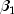,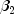, ...,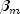are the regression coefficients for the covariates, and the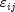's are independently distributed normal errors with mean zero and variance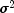. This model allows the regression function for each group to have different intercepts. However, the remaining m regression coefficients are the same for each group, i.e., the regression functions are parallel.

In practice, sometimes the regression functions are not parallel. In addition to estimates for the model assuming parallelism (parallel regression planes), `ANCOVA` computes estimates and summary statistics for the separate regressions of each group. These estimates can be examined using the methods `getCoefficientTables` and ``` getANOVATables```.

Estimates for the's and,, ...,in the model assuming parallelism are returned using the method `getModelCoefficients`. Summary statistics are also computed for this model and returned by the `compute` method.

The adjusted group means, stored in the last column of xymean, are computed using the formula: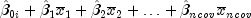where xymean is the matrix returned by `getMeans` and ncov is the number of covariates.

The estimated covariance between the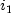-th and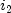-th adjusted group mean is given by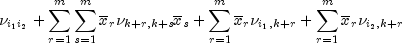where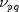is the entry in `covb[p-1][q-1]`, where `covb` is returned by `getVarCovCoefficients` and is the estimated covariance between the p-th and q-th estimated coefficients in the regression function.

Example 1, Example 2, Serialized Form
• ### Constructor Summary

Constructors
Constructor and Description
```ANCOVA(double[][] responses, double[][][] covariates)```
Constructs a one-way classification model with covariates.
• ### Method Summary

Methods
Modifier and Type Method and Description
`double[]` `compute()`
Performs one-way analysis of covariance assuming parallelism and returns an array containing the parallelism tests for the one-way analysis of covariance.
`double[]` `getAdjustedANOVA()`
Returns the partial sum of squares for the one-way analysis of covariance.
`double[]` `getANCOVA()`
Returns an array containing the one-way analysis of covariance assuming parallelism.
`double[][]` `getANOVATables()`
Returns a matrix of size ngroup by 15 containing the analysis of variance tables for each linear regression model fitted separately to each treatment group.
`double[][]` `getCoefficientTable(int group)`
Returns a matrix of size ncov + 1 by 4 containing statistics for a linear regression model fitted separately for each of the ngroup treatment groups.
`double[][][]` `getCoefficientTables()`
Returns an array containing statistics for a linear regression model fitted separately for all ngroup treatments.
`double[][]` `getMeans()`
Returns a matrix containing the unadjusted means for the covariates and the response variate and the means for the response variate adjusted for the covariates.
`double[][]` `getModelCoefficients()`
Returns a matrix containing statistics for the regression coefficients for the model assuming parallelism.
`int` `getNumberOfMissing()`
Returns the number of cases with missing values in ```covariates ``` or `responses`.
`double[][]` `getR()`
Returns the R matrix from the QR decomposition.
`double[][]` `getVarCovAdjustedMeans()`
Returns a matrix containing the estimated variances and covariances for the adjusted means assuming parallelism.
`double[][]` `getVarCovCoefficients()`
Returns a matrix containing the estimated variances and covariances for the coefficients returned using `getModelCoefficients`.
• ### Methods inherited from class java.lang.Object

`clone, equals, finalize, getClass, hashCode, notify, notifyAll, toString, wait, wait, wait`
• ### Constructor Detail

• #### ANCOVA

```public ANCOVA(double[][] responses,
double[][][] covariates)```
Constructs a one-way classification model with covariates.
Parameters:
`responses` - a `double` matrix containing the responses. Each row in `responses` corresponds to a treatment group. Each row of `responses` can contain a different number of observations. There must be at least two groups (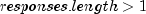).
`covariates` - is a three-dimensional `double` array containing the covariates. The first dimension corresponds to the number of covariates (consider each element an individual covariate matrix or ``` covariates.length``` = number of covariates). Each row in `covariates[i]` corresponds to a treatment group. There must be the same number of rows, for each covariate, as there are in the responses matrix (```covariates[i].length = responses.length```). There must be at least one covariate (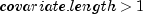). Each row of `covariates[i]` must contain the same number of elements as the corresponding row in `responses`
(``` covariates[i][j].length = responses[j].length```).
• ### Method Detail

• #### compute

`public double[] compute()`
Performs one-way analysis of covariance assuming parallelism and returns an array containing the parallelism tests for the one-way analysis of covariance.
Returns:
a `double` array containing the parallelism tests for the one-way analysis of covariance organized as follows:

 `index` `Description` 0 Extra degrees of freedom for model not assuming parallelism. 1 Degrees of freedom for error for model not assuming parallelism. 2 Degrees of freedom for error for model assuming parallelism. 3 Extra sum of squares for model not assuming parallelism. 4 Sum of squares for error for model not assuming parallelism. 5 Sum of squares for error for model assuming parallelism. 6 Mean square for index = 0. 7 Mean square for index = 1. 8 F statistic 9 p-value

`public double[] getAdjustedANOVA()`
Returns the partial sum of squares for the one-way analysis of covariance.
Returns:
a `double` array containing the partial sum of squares for the one-way analysis of covariance organized as follows:

 index Description 0 Degrees of freedom for groups after covariates. 1 Degrees of freedom for covariates after groups. 2 Sum of squares for groups after covariates. 3 Sum of squares for model (groups and covariates combined). 4 F-statistic for groups. 5 F-statistic for covariates. 6 p-value for groups. 7 p-value for covariates.

• #### getANCOVA

`public double[] getANCOVA()`
Returns an array containing the one-way analysis of covariance assuming parallelism.
Returns:
a `double` array of length 15 containing the following values:

 index ANCOVA Table Value 0 Degrees of freedom for model 1 Degrees of freedom for error 2 Total degrees of freedom 3 Sum of squares for model 4 Sum of squares for error 5 Total sum of squares 6 Model mean square 7 Error mean square 8 F statistic 9 p-value 10 R-squared (in percent) 11 Adjusted R-squared (in percent) 12 Estimated standard deviation of the model error 13 Mean of the response (dependent variable) 14 Coefficient of variation (in percent)

• #### getANOVATables

`public double[][] getANOVATables()`
Returns a matrix of size ngroup by 15 containing the analysis of variance tables for each linear regression model fitted separately to each treatment group.
Returns:
a `double` matrix containing the analysis of variance tables for each linear regression model fitted separately to each treatment group. The 15 values in the i-th row are for treatment group i organized as follows:

 index Description 0 Degrees of freedom for model 1 Degrees of freedom for error 2 Total degrees of freedom 3 Sum of squares for model 4 Sum of squares for error 5 Total sum of squares 6 Model mean square 7 Error mean square 8 F statistic 9 p-value 10 R-squared (in percent) 11 Adjusted R-squared (in percent) 12 Estimated standard deviation of the model error 13 Mean of the response (dependent variable) 14 Coefficient of variation (in percent)

• #### getCoefficientTable

`public double[][] getCoefficientTable(int group)`
Returns a matrix of size ncov + 1 by 4 containing statistics for a linear regression model fitted separately for each of the ngroup treatment groups.
Parameters:
`group` - an `int` specifying the group that regression statistics will be retreived for.
Returns:
a `double` matrix containing statistics for a ``` group```. Each row corresponds to the model coefficients. For row = 0, the statistics relate to the intercept in the regression model. For row = 1, 2, ..., ncov, the statistics relate to the slopes for the covariates. The column dimension corresponds to the row described for `getModelCoefficients` as follows:

 Column Description 0 Coefficient estimate. 1 Estimated standard error of the estimate. 2 t-statistic. 3 p-value.

• #### getCoefficientTables

`public double[][][] getCoefficientTables()`
Returns an array containing statistics for a linear regression model fitted separately for all ngroup treatments.
Returns:
a `double[][][]` array containing statistics for a linear regression model fitted separately for each of the ngroup treatment groups. The 3 dimensional array organized with ngroup rows, ncov + 1 columns, and depth of 4. Each row corresponds to one of the ngroup treatment groups. Each column corresponds to the model coefficients. For column = 0, the statistics relate to the intercept in the regression model. For column = 1, 2, ..., ncov, the statistics relate to the slopes for the covariates. The depth dimension corresponds to the columns described for ``` getModelCoefficients``` as follows:

 Column Description 0 Coefficient estimate. 1 Estimated standard error of the estimate. 2 t-statistic. 3 p-value.

• #### getMeans

`public double[][] getMeans()`
Returns a matrix containing the unadjusted means for the covariates and the response variate and the means for the response variate adjusted for the covariates.
Returns:
a `double` matrix of size ngroup + 1 by ncov + 3 containing the unadjusted means for the covariates and the response variate and the means for the response variate adjusted for the covariates. Each row for i = 0, 1, ..., ngroup - 1 corresponds to a group. Row ngroup contains overall statistics. The means are organized in columns as follows:

 Column Description 0 Number of non-missing cases 1 thru ncov Covariate means. ncov + 1 Response mean. ncov + 2 Response mean adjusted assuming parallelism.

• #### getModelCoefficients

`public double[][] getModelCoefficients()`
Returns a matrix containing statistics for the regression coefficients for the model assuming parallelism.
Returns:
a `double` matrix of size ngroup + ncov by 4 containing statistics for the regression coefficients for the model assuming parallelism. Each row corresponds to a coefficient in the model. For i = 0, 1, ..., ngroup - 1, row `i` is for the y intercept for the i -th group. The remaining ncov rows are for the covariate coefficients. The statistics in the columns are organized as follows:

 Column Description 0 Coefficient estimate. 1 Estimated standard error of the estimate. 2 t-statistic. 3 p-value.

• #### getNumberOfMissing

`public int getNumberOfMissing()`
Returns the number of cases with missing values in ```covariates ``` or `responses`. Cases with any missing values are not used in the analysis.
Returns:
an `int` scalar value indicating the number of cases with missing values in `covariates` or ``` responses```.
• #### getR

`public double[][] getR()`
Returns the R matrix from the QR decomposition. The R matrix is from the regression assuming parallelism.
Returns:
a `double` matrix of size ngroup + ncov by ngroup + ncov which contains the R from the QR decomposition. The R matrix is from the regression assuming parallelism.

`public double[][] getVarCovAdjustedMeans()`
Returns a matrix containing the estimated variances and covariances for the adjusted means assuming parallelism.
Returns:
a `double` matrix of size ngroup by ngroup containing the estimated variances and covariances for the adjusted means assuming parallelism.
• #### getVarCovCoefficients

`public double[][] getVarCovCoefficients()`
Returns a matrix containing the estimated variances and covariances for the coefficients returned using `getModelCoefficients`.
Returns:
a `matrix` of size ngroup + ncov by ngroup + ncov containing the estimated variances and covariances for the coefficients returned using ``` getModelCoefficients```.
JMSLTM Numerical Library 7.2.0